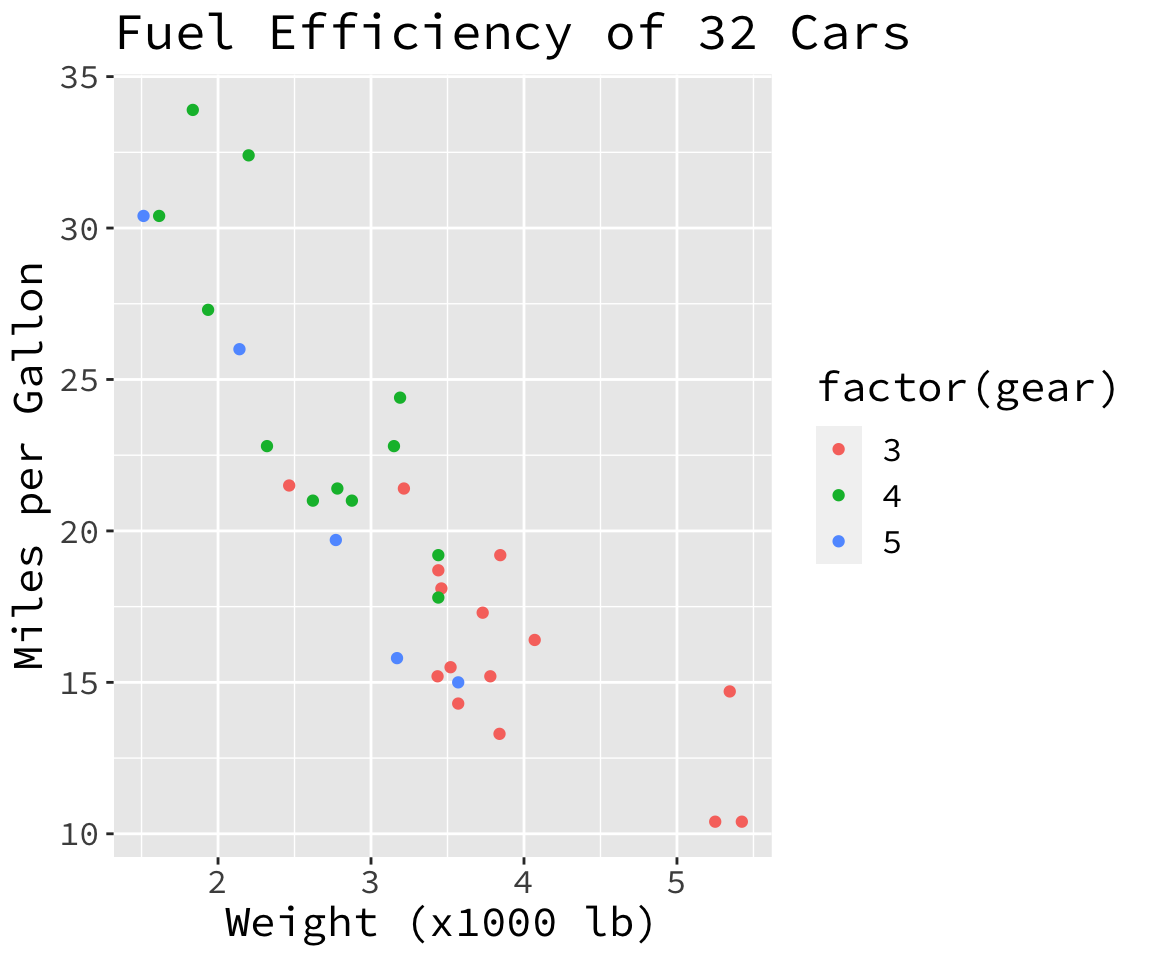Sometimes you want to use some custom fonts in your ggplot2 graphics. Years ago there was a good article in [revolutionanalytics.com].

But this article states that if you want to use otf-fonts instead of ttf-fonts you have to use some experimental packages:

 ``````1 2 3 `````` ``````library(devtools) install_github('wch/Rttf2pt1') install_github('wch/extrafont') ``````

But as time goes by the evolution of R-packages progresses. So now you can use otf-fonts in ggplot2 graphics. I’m using Source Code Pro as custom font because I can identify this font really good because of its “zeros”.

 ``````1 2 3 4 5 6 `````` ``````library(ggplot2) ggplot(mtcars, aes(x=wt, y=mpg, color=factor(gear))) + geom_point() + ggtitle('Fuel Efficiency of 32 Cars') + xlab('Weight (x1000 lb)') + ylab('Miles per Gallon') + theme(text=element_text(size=16, family='Source Code Pro')) ``````